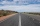# Right triangle - math word problems

1. Diamond and diagonalsA diamond has diagonals f = 8 cm and g = 6 cm long. How long is this diamond perimeter? (Calculate it!)
2. Prism 4 sidesThe prism has a square base with a side length of 3 cm. The diagonal of the sidewall of the prism/BG/is 5 cm. Calculate the surface of this prism in cm square and the volume in liters
3. Pyramid heightFind the volume of a regular triangular pyramid with edge length a = 12cm and pyramid height h = 20cm.
4. Castle towerThe castle tower has a cone-shaped roof with a diameter of 10 meters and a height of 8 meters. Calculate how much m² of coverage is needed to cover it if we must add one-third for the overlap.
5. The diagram 2The diagram shows a cone with slant height 10.5cm. If the curved surface area of the cone is 115.5 cm2. Calculate correct to 3 significant figures: *Base Radius *Height *Volume of the cone
6. The sides 2The sides of a trapezoid are in the ratio 2:5:8:5. The trapezoid’s area is 245. Find the height and the perimeter of the trapezoid.
7. Find parametersFind parameters of the circle in the plane - coordinates of center and radius: ?
8. Isosceles triangle 8If the rate of the sides an isosceles triangle is 7:6:7, find the base angle correct to the nearest degree.
9. LighthouseMarcel (point J) lies in the grass and sees the top of the tent (point T) and behind it the top of the lighthouse (P). | TT '| = 1.2m, | PP '| = 36m, | JT '| = 5m. Marcel lies 15 meters away from the sea (M). Calculate the lighthouse distance from the sea
10. Cable carFind the elevation difference of the cable car when it rises by 67 per mille and the rope length is 930 m.
11. Spherical cap 4What is the surface area of a spherical cap, the base diameter 20 m, height 2.5 m? Calculate using formula.
12. Horizontal distanceThe road has a gradient of 8%. How many meters will the road rise on a horizontal distance of 400m?
13. Pile of sandA large pile of sand has been dumped into a conical pile in a warehouse. The slant height of the pile is 20 feet. The diameter of the base of the sand pile is 31 feet. Find the volume of the pile of sand.
14. CincinnatiA map is placed on a coordinate grid. Cincinnati located at (5,4) and San Diego is located at (-10, -3). How far apart is Cincinnati from San Diego on the map? Round to the nearest tenth.
15. Right triangleLadder 16 feet reaches up 14 feet on a house wall. The 90-degree angle at the base of the house and wall. What are the other two angles or the length of the leg of the yard?
16. Spherical capPlace a part of the sphere on a 4.6 cm cylinder so that the surface of this section is 20 cm2. Determine the radius r of the sphere from which the spherical cap was cut.
17. Prism diagonalThe body diagonal of a regular square prism has an angle of 60 degrees with the base, the edge length is 10 cm. What is the volume of the prism?
18. RectangleThere is a rectangle with a length of 12 cm and a diagonal 8 cm longer than the width. Calculate the area of rectangle.
19. Triangular pyramidWhat is the volume of a regular triangular pyramid with a side 3 cm long?
20. KLM triangleFind the length of the sides of the triangle KLM if m = 5cm height to m = 4.5 cm and size MKL angle is 70 degrees.

Do you have an interesting mathematical word problem that you can't solve it? Enter it, and we can try to solve it.

We will send a solution to your e-mail address. Solved examples are also published here. Please enter the e-mail correctly and check whether you don't have a full mailbox.

Please do not submit problems from current active competitions such as Mathematical Olympiad, correspondence seminars etc...